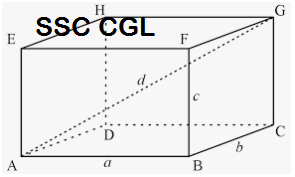# How to tackle Trigonometry questions in SSC CGL exam

Trigonometry questions are very important and among the most commonly asked questions in the SSC CGL examination. These questions might seem difficult at first but once known the correct way to tackle them then can be easily solved within fraction of seconds.

Trigonometry has its relevance in both Tier 1 and Tier 2 of SSC CGL exam. The questions that are basically asked under this section can be subdivided into the questions from various parts like questions based on triangles and their properties; questions based on values of sin, cos, tan, cosec, sec and cot; questions based on the relation between sin, cos, tan, sec, cosec and cot and the questions based on heights and distance.

### How to tackle trigonometry questions in SSC CGL Exam?

1. Go through the basic details that are needed to attempt this topic. Grasp all the important points and formulas.
For example:
For solving this question, one needs to be aware of the basic formulas required.

As, we know , where n is an odd number.
So,

1. Trigonometry is basically a mind game. The difficult question might have the easiest solution. So, try to solve first by visualizing and find out what can be eliminated at first and how the lengthy question can be made shorter without using pen and paper.
For example:
So, by visualizing we can see that are opposite pairs. Thus, they cancel out each other. Also,
So,
2. There is a surety that at least one question would be asked from the sub-topic: heights and distances. So, prepare for the topics like angle of elevations, angle of depression, questions on ships, towers, flag posts, ladder, wall etc. This is sure shot thing and one question would be asked out of the above mentioned parts.
For example:
A pole of height 15 meters has a shadow of length equal to the height of the pole. Find the angle of elevation of the sun.

Let, AB be the length of the pole and BC be its shadow.
Given, AB = BC = 15m
Let x be the angle of elevation of the sun.
So,
So,
So, x = 45

Such questions are asked from heights and distances with variations. Go through what is given. Draw a figure accordingly and then calculate what is being asked. Sometimes it can directly be solved just with the help of sketch. So, try to save time in every step.4. Try solving questions by evaluating the options. Sometimes reverse process will help you in saving time with accurate results. So, if questions can be solved with the help of values given in the options then don’t waste time in solving the questions unnecessarily. Apply your common sense.

5. Cramming ‘n’ number of formulas will lead you nowhere. Three things should be kept in mind i.e. Practice! Practice! And Practice! Learning by doing will give you zero error and will help you getting command over this area.

For solving such questions, only few identities are needed. And the rest could be derived. So, don’t cram each and everything. Learn what couldn’t be derived.

1. Trigonometry questions asked in SSC CGL are mostly based on common identities rather than the hard core trigonometric formulas that you might have studied before. So, first identify if any identity could replace the given equation and then try solving further.
2. Two types of questions can be asked, i.e., pure identity based or the questions based on applications. So, equal importance must be given to both type of questions and students should prepare accordingly. Also, don’t cram all the formulas. Learn only the basic formulas thoroughly and the rest can be derived.
For example:

Such questions are based on basic identities.
As, we know , where n is an odd number.
So,

So, trigonometry is a very important and interesting topic if studied properly.  Leaving this part completely will not only take away your few marks but at the same time, it will also lag you behind hundreds of aspirants. Even one mark can make a difference, so, risking 5 – 10 questions will definitely affect your overall results in the SSC CGL Exam So, be prepared for this topic well and try to attempt all the questions on this topic with 100% accuracy.

You can also download SSC CGL preparation app from Google Play Store. It provides free study notes, quizzes, mock test, online practice set, question papers, notifications, & more.